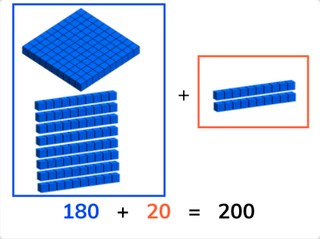# Adding to a hundreds number

Students learn to add to a multiple of 100 within 1,000.8,000 schools use Gynzy92,000 teachers use Gynzy1,600,000 students use Gynzy

## General

Students learn to add to a multiple of 100.

1.NBT.C.4

## Relevance

Discuss with students that it is important to add numbers to a hundreds number, so you can determine a total.

## Introduction

Show the money on the interactive whiteboard and tell students that they must add to the amounts to compose 100 or 200. The students must determine how many more must be added. Next tell students to decompose the given numbers. Ask students to write down their answers and hold them up on a sheet of paper or interactive whiteboard.

## Development

Check that students are able to add to a hundreds number by asking the following questions:
- What is a useful way to add to a hundreds number?
- How do you solve 385 + _ = 400?

## Guided practice

Students are given problems in which they must add two numbers to a hundreds number with visual support. They must make jumps on a number line as well as simply add the numbers. They are also given story problems and problems in which they must determine what the missing addend is.

## Closing

Discuss with the students that it is important to be able to add to a hundreds number, so you can determine a total. Show the number line on the interactive whiteboard and ask students to discuss how they would jump to the given total. Then discuss the problem as a class and show some possible jumps on the number line. Next ask students to determine what the number hidden below the paint splatters are. After giving them time to think, ask students to give their answers by turn. Ask them to explain their strategy. Delete the paint splatters away to show the correct answers.

## Teaching tips

If students have difficulty adding to a hundreds number, they can be supported by making use of a number line as visual support. Remind students that they can make different jumps on the number line to reach the total.

### The online teaching platform for interactive whiteboards and displays in schools

• Save time building lessons

• Manage the classroom more efficiently

• Increase student engagement